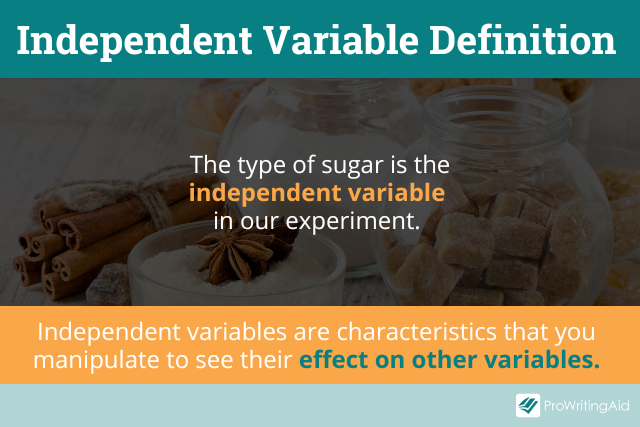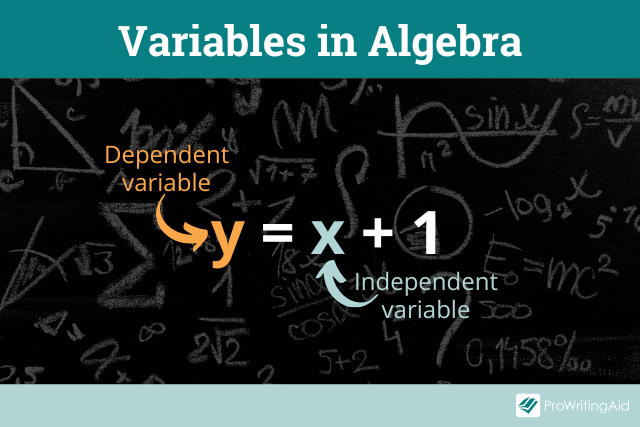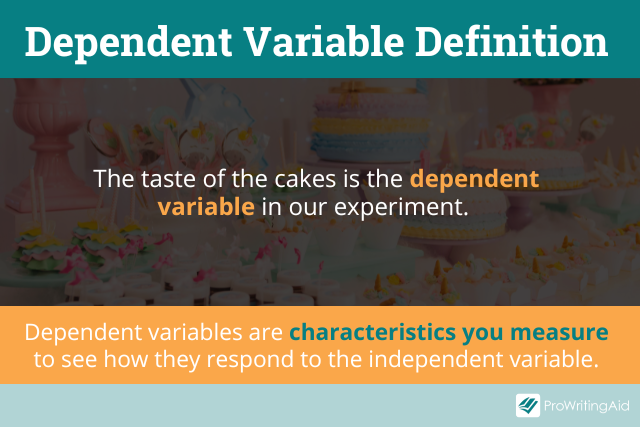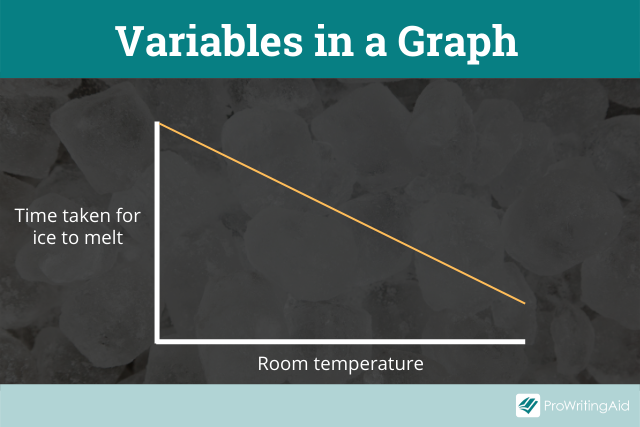# Independent vs Dependent Variables

If you’ve ever done a science experiment or solved an algebra equation, you’ve probably worked with variables.

It’s important to remember the difference between independent and dependent variables, because they’re two key variables in both math and science.

Essentially, you can think of the independent variable as the factor you manipulate, and the dependent variable as the factor you measure.

## What Is an Independent Variable?

An independent variable is a characteristic that you manipulate to see its effect on dependent variables.

It’s called an independent variable because you can set its value independently of everything else in the experiment.You can decide on the value of this variable, and all other variables in the experiment will depend on that value.

The independent variable is also known as the manipulated variable or the input variable.

In algebra, the independent variable is the variable you can set to anything.

For example, in the equation “y = x + 1,” x is the independent variable because it’s the input value. You can set it to anything you want, and then solve for y.Let’s consider an experimental example where you want to find out how much your walking pace affects your heart rate.

To test this out, you might measure your heart rate while you’re walking at various speeds: slow, medium, and fast.

In this case, the independent variable is your walking pace, because that’s the factor you’re changing to see how pace affects your heart rate.

## What Is a Dependent Variable?

A dependent variable is the characteristic you measure to see how it responds to the independent variable.The term dependent variable makes sense because the value of this variable is dependent on the independent variable. The dependent variable is also known as the responding variable or the output variable.

You need to measure the value of your dependent variable to find out the results of any experiment.

In contrast, you don’t need to measure the value of your independent variablebecause you already know what its value is—after all, you set it yourself.

Consider the algebraic equation we discussed earlier: “y = x + 1.” In this case, y is the dependent variable because it depends on x. Its value will always be 1 higher than x.

The dependent variable is your heart rate, because your heart rate depends on how fast you’re walking. That’s the outcome you want to measure in your experiment.

## Independent and Dependent Variables in Science and Research

Remembering the difference between independent and dependent variables is crucial for scientific research.

Scientists and researchers conduct experiments by choosing an independent variable and setting it to a range of different values.

They then measure how the changing independent variable affects the dependent variables.

It’s generally best to only manipulate one independent variable, so you don’t have any other factors at play. Limiting yourself to one independent variable ensures you can have a controlled experiment.

For example, you wouldn’t want to measure your heart rate while walking at different speeds, singing different types of music, and eating different types of food all at the same time.

Instead, you should change those variables one at a time so you can isolate their effects.

However, it’s okay to have more than one dependent variable outcomes if you want to measure multiple multiple.

For example, you could measure both your heart rate and your blood pressure while walking at different speeds, to see which one is affected more.

When researchers plot experimental results on a graph, it’s standard convention to have the independent variable on the horizontal axis, or x-axis, and the dependent variable on the vertical axis, or y-axis.

Doing this allows readers to clearly see how one of these key variables affects the other.## Examples Showing the Difference Between Independent vs Dependent Variables

Let’s look at some more examples of independent and dependent variables in science experiments.

### Weighted Paper Airplanes Example

Say you want to answer the following research question: “How does the weight of a paper airplane affect how far it flies?”

To figure out the answer, you’d probably want to make a paper airplane, throw it, and measure how far it can fly.

Then, you would add some weight to the airplane—say, by taping a coin to the bottom—and throw it again to see the new flight distance.

In this experiment, the independent variable is how much the paper airplane weighs, because this is the characteristic you’re manipulating.

The dependent variable is the distance the paper airplane flies, because this is the characteristic you’re measuring.

### Musical Test Scores Example

Now imagine you want to answer the following research question: “How does listening to different types of music affect math test scores?”

If you’re a professional researcher with access to an academic institution, you might be able to gather a group of students willing to volunteer.

You can put one group of students in a room with classical music, put another in a room with pop music, and another in a room with rock ‘n’ roll music.

Then, you’d ask them all to take the same math test. It’s important not to give some of them algebra tests and others calculus tests because that would be another independent variable, which might confuse the results.

After they’re done, you would collect the average test score in each room. That way, you can see how the different music affected the scores in each room.

In this experiment, the independent variable is the type of music played in each room, because that’s the factor that you’ve deliberately manipulated.

The dependent variable is the average exam score in each room, because that’s the outcome you want to find out.

### Favorite Ice Cream Example

Finally, let’s look at a very simple experiment you can conduct at home.

In this experiment, the research question is: “What brand of vanilla ice cream do you think tastes best?”

To conduct this experiment, you’ll need to buy several different brands of vanilla ice cream.

It doesn’t have to be vanilla, but it is important to standardize to one flavor to avoid any confounding variables.

Then, you can try a spoonful of ice cream from each brand, and rate how much you like it on a scale of 1 to 10.

In this experiment, the independent variable is the brand of ice cream, because that’s the factor you’ve changed on purpose.

The dependent variable is how much you like each type of the ice cream, because that’s the truth you’re trying to discover.

I hope these examples helped give you a better sense of the difference between independent and dependent variables.

Now you can use these important terms to improve your scientific knowledge and academic writing skills.

### Take your writing to the next level: